# JavaScript 法術合集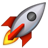​前期回顧​

Vue項目實戰 —— 後臺管理系統( pc端 ) 第三篇_0.活在風浪裡的博客-CSDN博客mock模擬數據、折線圖、柱狀圖、餅圖，一遍就懂！！~
https://blog.csdn.net/m0_57904695/article/details/124861409″ >目錄

JavaScript 語法之創建對象的三種方法

1、通過內置的構造函數創建對象

2、通過字面量創建對象

3、通過構造函數

​ 獲取後臺接口的前五條數據

JavaScript 語法之遞歸

遞歸事例2

2.1、for循環 求 1-100的和

2.2、  遞歸 求1-100的和

JavaScript 語法之創建對象的三種方法 1、通過內置的構造函數創建對象

``    const obj = new Object();    obj.name = '張三';    obj.age = 18;    obj.fn = function() {        console.log('我是一個方法');    }    console.log(obj);``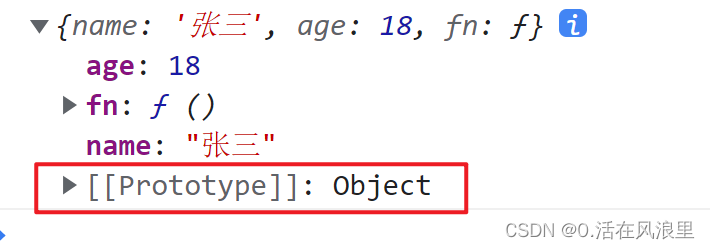2、通過字面量創建對象

``    let obj = {        name: '我隻會心疼哥哥',        age: 0,    }    console.log(obj)``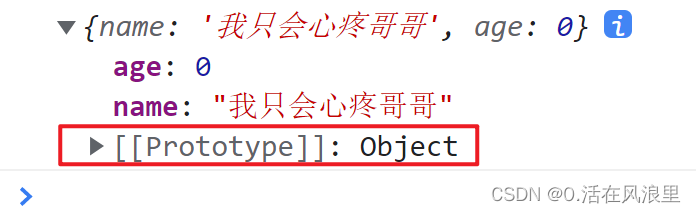3、通過構造函數

``    function Person(name, age) { //  構造函數         this.age = age;        this.name = name;        this.run = function() {            console.log('我叫' + this.name + '今年' + this.age + '我跑步可快瞭');        }    }    // prototype 原型    Person.prototype.say = function() { //  把say  方法  掛載到Person 原型對象        console.log('我叫' + this.name + '今年' + this.age); //引引加加 加加裡面是變量    }    Person.prototype.eat = function() {        console.log(this.name + '吃瞭一頓飯');    }    var obj = new Person('你不會怪我吧', '28'); // 實例化對象 // new 創建對象 傳參     console.log(obj);    obj.say();    obj.eat();    obj.run(); //實例過後才可以調方法和屬性``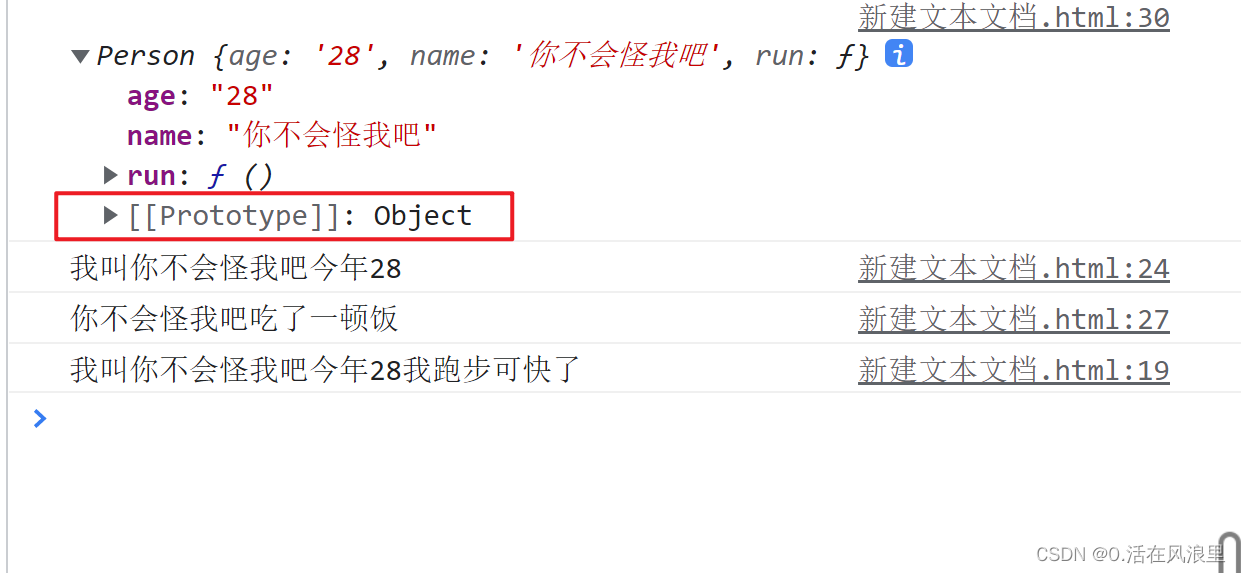獲取後臺接口的前五條數據

`` axios.get("/api/home/xiaoguotu").then((res) => {      // console.log(res.data.data.list)      this.list = res.data.data.list;      // 將接口的前5條取出來      for (let v = 0; v < 5; v++) { //0 1 2 3 4 5        this.list.push(this.list[v]);      }      console.log(this.slist);    });``

JavaScript 語法之遞歸

遞歸的概念  :

註意： 函數內部調用自己， 必須要有一個退出條件，條件寫在開始位置，不要寫在結束位置，否則會無限循環 函數的調用，可以放在循環裡面， 也可以放在函數裡面

為何報錯？

如果你在遞歸中，遞歸的變量越來越不接近遞歸結束條件，那麼就會報出 StackOverflowError 這個錯誤，歸根到底就是JVM中棧的空間已經不夠容納遞歸所需的空間， 所以報錯。

如果你遞歸的數量十分龐大， 那麼也會報出 StackOverflowError。

``    let day = 0;    fn()    function fn() {        day++;        console.log('你這個老六真的夠瞭，第' + day + '天,還不放假');        if (day < 2) {            fn()        }    }``

打個斷點 day===2直接退出函數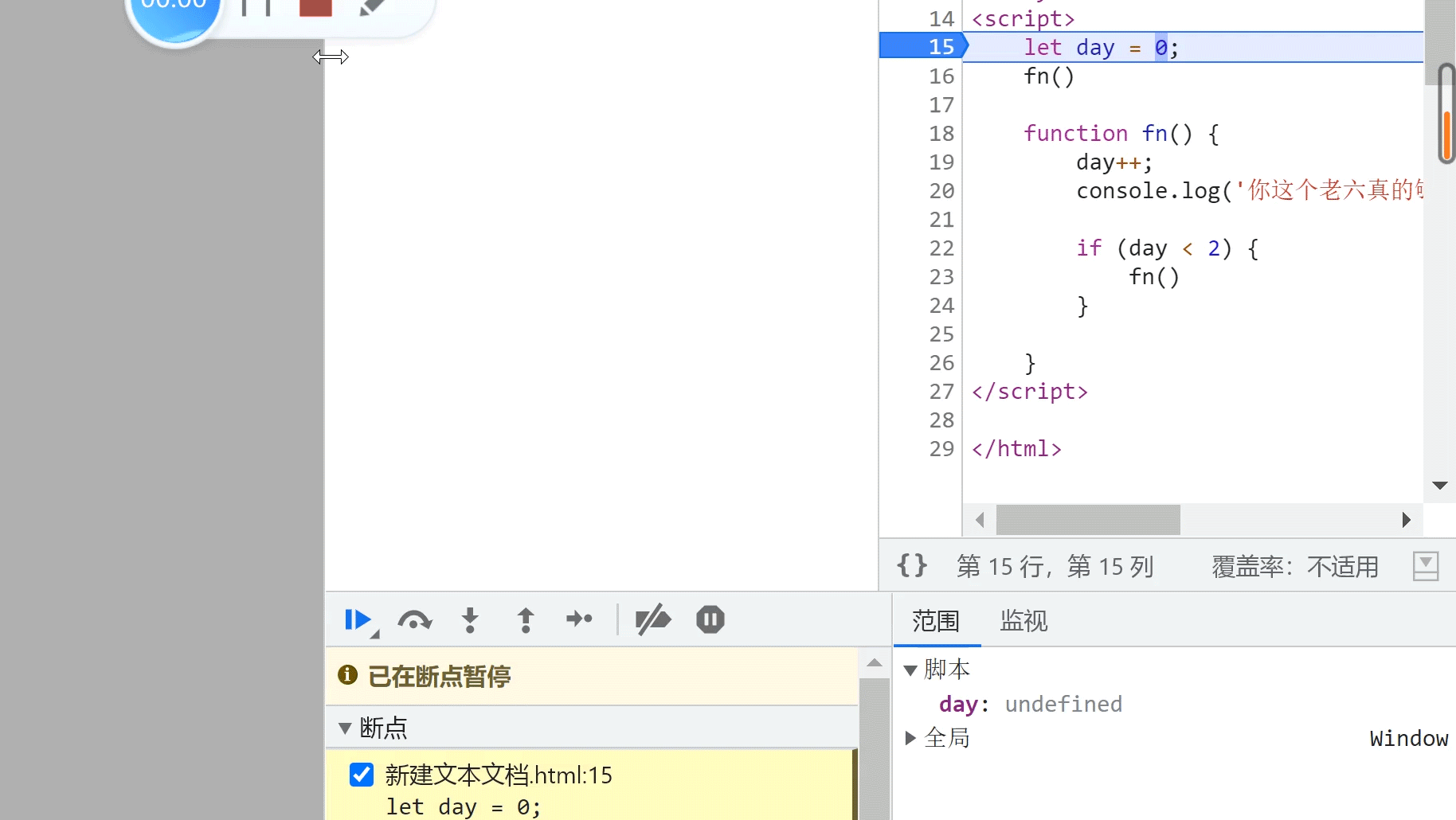遞歸事例2 2.1、for循環 求 1-100的和

``    var sum = 0;    for (var i = 1; i <= 100; i++) {        sum += i; // 0+1 0+2 0+3 ...    }    console.log(sum);``

2.2、  遞歸 求1-100的和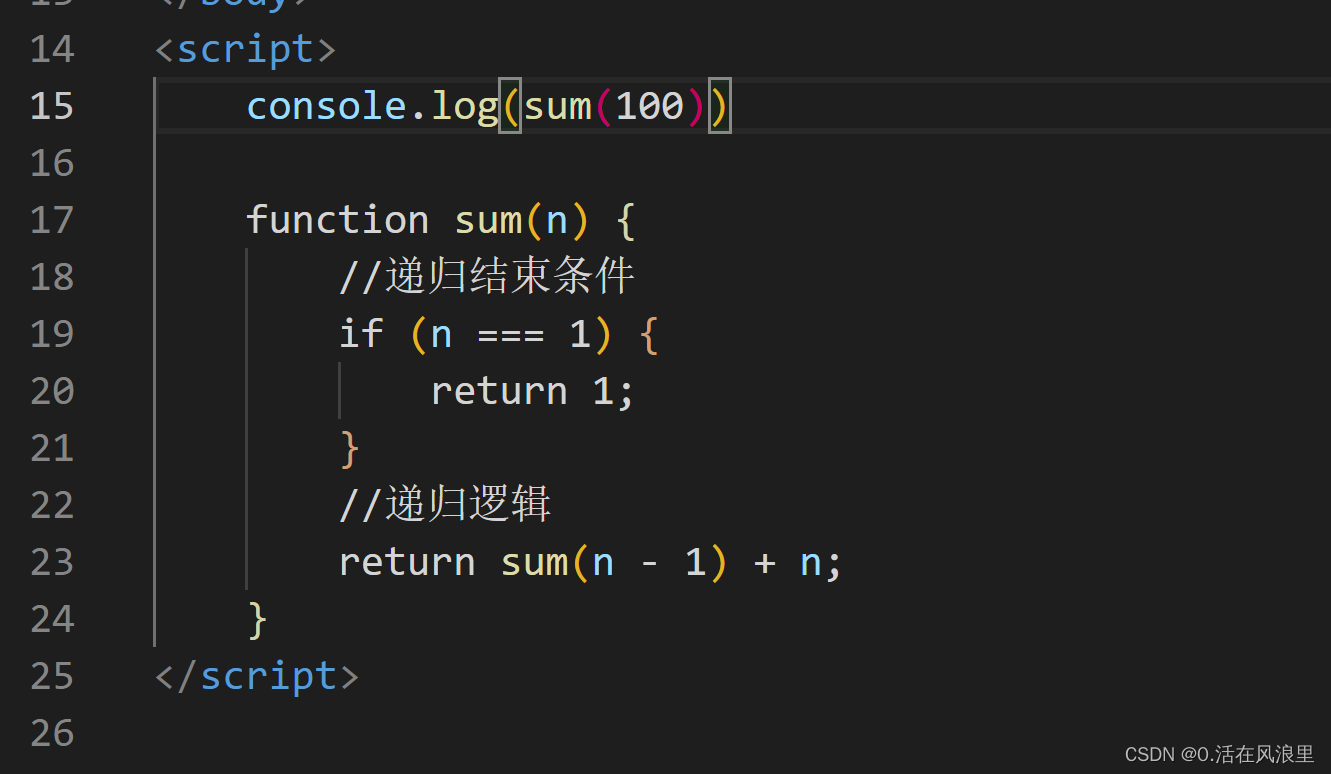先求得1到2的和

``    console.log(sum(2)); //現在n===2    function sum(n) {        return n + n            //2+2=4 因為求1到2的和現在n是2要到1才行，所以-1變成 1+2=3，對吧，那麼代碼就得改成        }----------------------------------------------------------------------------------- console.log(sum(3));     function sum(n) {      return (n-1) + n      // 如果求1-3的和還的讓這個函數調用2次才行（3-1 2-1），需要讓它自調用，代碼得改    }-----------------------------------------------------------------------------------  console.log(sum(3));    function sum(n) {        return sum(n - 1) + n; //遞歸調用     }``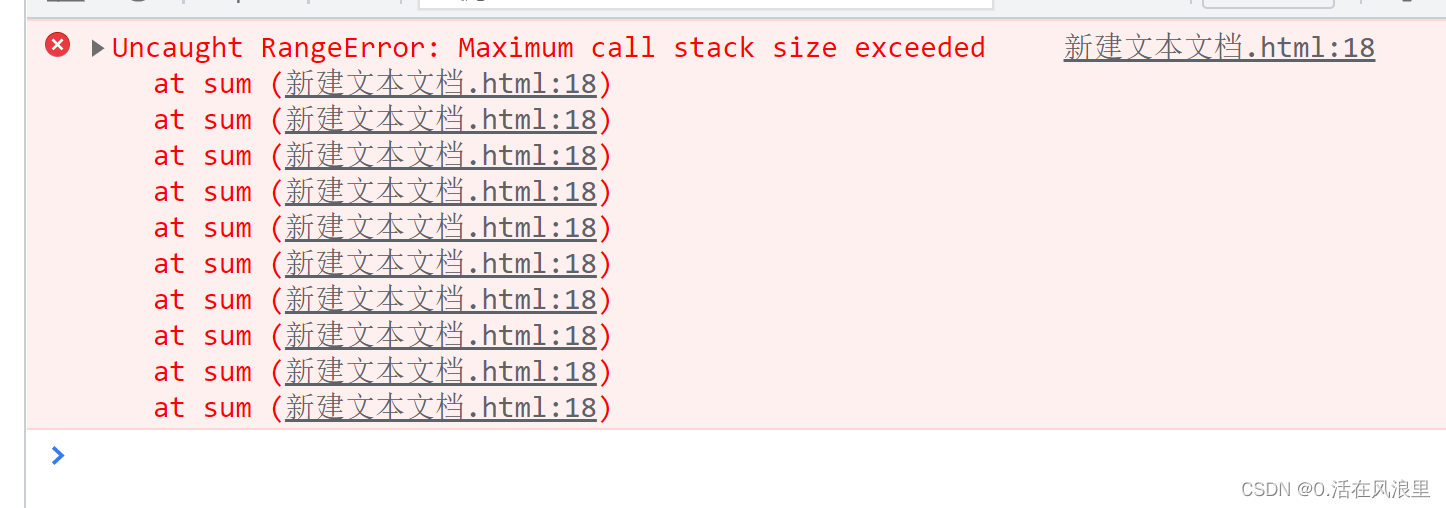``    console.log(sum(100))    function sum(n) {        //遞歸結束條件        if (n === 1) {            return 1;        }        //遞歸邏輯        return sum(n - 1) + n;    }``

2022/5/20 19:32